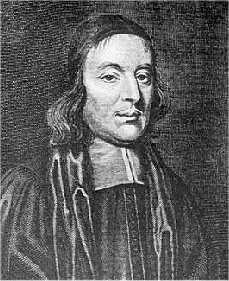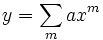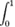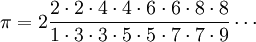# Lit2Go

## A Short Account of the History of Mathematics

### by W.W. Rouse Ball

#### John Wallis

• Year Published: 1908
• Language: English
• Country of Origin: England
• Source: Ball, W.W.R. (1908). A Short Account of the History of Mathematics. London, New York: Macmillan.
• Flesch–Kincaid Level: 12.0
• Word Count: 1,759
• Genre: History
• Keywords: biographyJohn Wallis was born at Ashford on November 22, 1616, and died at Oxford on October 28, 1703. He was educated at Felstead school, and one day in his holidays, when fifteen years old, he happened to see a book of arithmetic in the hands of his brother; struck with curiosity at the odd signs and symbols in it he borrowed the book, and in a fortnight, with his brother's help, had mastered the subject. As it was intended that he should be a doctor, he was sent to Emmanuel College, Cambridge, while there he kept an "act" on the doctrine of the circulation of the blood; that was said to have been the first occasion in Europe on which this theory was publicly maintained in a disputation. His interests, however, centred on mathematics.

He was elected to a fellowship at Queens' College, Cambridge, and subsequently took orders, but on the whole adhered to the Puritan party, to whom he rendered great assistance in deciphering the royalist despatches. He, however, joined the moderate Presbyterians in signing the remonstrance against the execution of Charles I., by which he incurred the lasting hostility of the Independents. In spite of their opposition he was appointed in 1649 to the Savilian chair of geometry at Oxford, where he lived until his death on October 28, 1703. Besides his mathematical works he wrote on theology, logic, and philosophy, and was the first to devise a system for teaching deaf-mutes. I confine myself to a few notes on his more important mathematical writings. They are notable partly for the introduction of the use of infinite series as an ordinary part of analysis, and partly for the fact that they revealed and explained to all students the principles of the new methods of analysis introduced by his contemporaries and immediate predecessors.

In 1655 Wallis published a treatise on conic sections in which they were defined analytically. I have already mentioned that the Géométrie of Descartes is both difficult and obscure, and to many of his contemporaries, to whom the method was new, it must have been incomprehensible. This work did something to make the method intelligible to all mathematicians: it is the earliest book in which these curves are considered and defined as curves of the second degree.

The most important of Wallis's works was his Arithmetica Infinitorum, which was publishd in 1656. In this treatise the methods of analysis of Descartes and Cavalieri were systematised and greatly extended, but their logical exposition is open to criticism. It at once became the standard book on the subject, and is constantly referred to by subsequent writers. It is prefaced by a short tract on conic sections. He commences by proving the law of indices; shews that x0, x-1, x-2 ... represents 1, 1/x, 1/x²...; that x1/2 represents the square root of x, that x2/3 represents the cube root of x2, and generally that x-n represents the reciprocal of xn, and that xp/q represents the qth root of xp.

Leaving the numerous algebraical applications of this discovery he next proceeds to find, by the method of indivisibles, the area enclosed between the curve y = xm, the axis of x, and any ordinate x = h; and he proves that the ratio of this area to that of the parallelogram on the same base and of the same altitude is equal to the ratio 1 : m + 1. He apparently assumed that the same result would be true also for the curve y = axm, where a is any constant, and m any number positive or negative; but he only discusses the case of the parabola in which m = 2, and that of the hyperbola in which m = -1: in the latter case his interpretation of the result is incorrect. He then shews that similar results might be written down for any curve of the formand hence that, if the ordinate y of a curve can be expanded in powers of the abscissa, x, its quadrature can be determined: thus he says that if the equation of the curve were y = x0 + x1 + x2 + ⋅⋅⋅ , its area would be x + 1/2 x2 + 1/3 x3 + ⋅⋅⋅. He then applies this to the quadrature of the curves y = (x - x2)0, y = (x - x2)1, y = (x - x2)2, y = (x - x2)3, etc. taken between the limits x = 0 and x = 1; and shews that the areas are respectively 1, 1/6, 1/30, 1/140, etc. He next considers curves of the form y = x-m and establishes the theorem that the area bounded by the curve, the axis of x, and the ordinate x = 1, is to the area of the rectangle on the same base and of the same altitude as m : m + 1. This is equivalent to finding the value ofx1/mdx. He illustrates this by the parabola in which m = 2. He states, but does not prove, the corresponding result for a curve of the form y = xp/q.

Wallis shewed considerable ingenuity in reducing the equations of curves to the forms given above, but, as he was unacquainted with the binomial theorem, he could not effect the quadrature of the circle, whose equation is y = (x - x2)1/2, since he was unable to expand this in powers of x. He laid down, however, the principle of interpolation. Thus, as the ordinate of the circle y = (x - x2)1/2 is the geometrical mean between the ordinates of the curves y = (x - x2)0 and y = (x - x2)1, it might be suppose that, as an approximation, the area of the semicircle(x - x2)1/2dx which is 1/8π might be taken as the geometrical mean between the values of(x - x2)0 dx and(x - x2)1 dx

that is, 1 and 1/6; this is equivalent to taking 4√2/3 or 3.26... as the value of π. But, Wallis argued, we have in fact a series 1, 1/6, 1/30, 1/140, ... and therefore the term interpolated between 1 and 1/6 ought to be chosen so as to obey the law of this series. This, by an elaborate method, which I need not describe in detail, leads to a value for the interpolated term which is equivalent to takingThe mathematicians of the seventeenth century constantly used interpolation to obtain results which we should attempt to obtain by direct analysis.

In this work also the formation and properties of continued fractions are discussed, the subject having been brought into prominence by Brouncker's use of these fractions.

A few years later, in 1659, Wallis published a tract containing the solution of the problems on the cycloid which had been proposed by Pascal. In this he incidentally explained how the principles laid down in his Arithmetica Infinitorum could be used for the rectification of algebraic curves; and gave a solution of the problem to rectify the semi-cubical parabola x³ = ay² which had been discovered in 1657 by his pupil William Neil. Since all attempts to rectify the ellipse and hyperbola had been (necessarily) ineffectual, it had been supposed that no curves could be rectified, as indeed Descartes had definitely asserted to be the case. The logarithmic spiral had been rectified by Torricelli, and was the first curved line (other than the circle) whose length was determined by mathematics, but the extension by Neil and Wallis to an algebraical curve was novel. The cycloid was the next curve rectified; this was done by Wren in 1658.

Early in 1658 a similar discovery, independent of that of Neil, was made by van Heuraët, and this was published by van Schooten in his edition of Descartes's Geometria in 1659. Van Heuraët's method is as follows. He supposes the curve to be referred to rectangular axes; if this be so, and if (x,y) be the coordinates of any point on it, and n be the length of the normal, and if another point whose co-ordinates are (x, η )   be taken such that η : h = n : y, where h is a constant; then, if ds be the element of the length of the required curve, we have by similar triangles ds : dx = n : y. Therefore h ds = η dx. Hence, if the area of the locus of the point (x, η) can be found, the first curve can be rectified. In this way van Heuraët effected the rectification of the curve y³ = ax² but added that the rectification of the parabola y² = ax is impossible since it requires the quadrature of the hyperbola. The solutions given by Neil and Wallis are somewhat similar to that given by van Heuraët, though no general rule is enunciated, and the analysis is clumsy. A third method was suggested by Fermat in 1660, but it is inelegant and laborious.

The theory of the collision of bodies was propounded by the Royal Society in 1668 for the consideration of mathematicians. Wallis, Wren, and Huygens sent correct and similar solutions, all depending on what is now called the conservation of momentum; but, while Wren and Huygens confined their theory to perfectly elastic bodies, Wallis considered also imperfectly elastic bodies. This was followed in 1669 by a work on statics (centres of gravity), and in 1670 by one on dynamics: these provide a convenient synopsis of what was then known on the subject.

In 1685 Wallis published an Algebra, preceded by a historical account of the development of the subject, which contains a great deal of valuable information. The second edition, issued in 1693 and forming the second volume of his Opera, was considerably enlarged. This algebra is noteworthy as containing the first systematic use of formulae. A given magnitude is here represented by the numerical ratio which it bears to the unit of the same kind of magnitude: thus, when Wallis wants to compare two lengths he regards each as containing so many units of length. This perhaps will be made clearer if I say that the relation between the space described in any time by a particle moving with a uniform velocity would be denoted by Wallis by the formula s = vt, where s is the number representing the ratio of the space described to the unit of length; while the previous writers would have denoted the same relation by stating what is equivalent to the proposition s1 : s2 = v1t1 : v2t2. It is curious to note that Wallis rejected as absurd the now usual idea of a negative number as being less than nothing, but accepted the view that it is something greater than infinity. The latter opinion may be tenable and not inconsistent with the former, but it is hardly a more simple one.